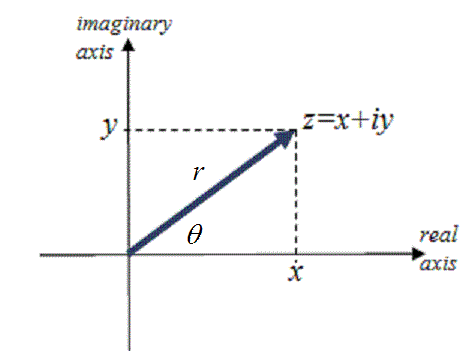# Section 4.4 Polar Form of a Complex Number

Section Objectives

1. Write complex numbers in polar (trigonometric) form.
2. Multiply and divide complex numbers in polar form.

### Polar or Trigonometric Form

The polar (or trigonometric) form of the complex number $z = x+yi$ is

$z=r(\cos \theta + i \sin \theta)$,

where $r=\sqrt{x^2+y^2}$ and $\tan \theta= y/x$. $r$ is the absolute value (or modulus) of $z$, and $\theta$ is called the argument of $z$. Normally, $\theta$ is restricted so that $0 \le \theta < 2\pi$.#### Examples

• Write $z=6-6i$ in polar form.

• Write $6+2i$ in polar form.

• Write $z=4(\cos 120^{\circ} + i \, \sin 120^{\circ})$ in standard form.

### Multiplication and Division in Polar Form

It is fairly easy to prove the following useful results.

Let $z_1=r_1(\cos \theta_1 + i \, \sin \theta_1)$ and $z_2=r_2(\cos \theta_2 + i \, \sin \theta_2)$.

• Let $z_1=2(\cos 120^{\circ} + i \, \sin 120^{\circ})$ and $z_2=8(\cos 330^{\circ} + i \, \sin 330^{\circ})$. Compute $z_1 z_2$ and $z_2/z_1$.